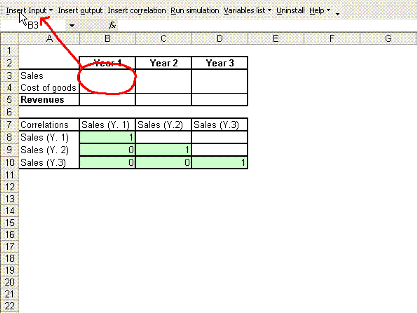Simulación 4.0

Spanish version

Simulacion4_0_Eng.xls (English version)

Simulacion4_0.xls (Spanish version)

Details

Simulación 4.0 was fully developed in VBA (Visual Basic for Applications) and it is compatible with Excel 97 and above. It has been created in order to bring a flexible simulation tool, easy to be used.

Features:

• 34 random number functions within the following distributions:

Continuous

 Arcsine Fisher Normal Uniform Beta Gamma Parabolic Weibull Cauchi Gamma Inverted Pareto Chi Square Gumbel Pearson’s type 6 Cosine Laplace Power Double Log. Logarithmic Rayleigh Exponential Logistic Students T Erlang LogNormal Triangular

Discrete

 Bernoulli Binomial Negative Hypergeometric Poisson Binomial Geometric Pascal Uniform (D)

(As long as the simulation is not running, these functions return the expected value)

Simulation with the Monte Carlo method, between 1 - 65,000 iterations.

Up to 150 Inputs and 20 Outputs.

Easy Block of inputs in order to run partial simulations.

• Definition of correlations between inputs.

• Determination of  one approximate distribution for a known data series.

Quick and easy install and uninstall.

Simulation results presented in a new Excel Workbook with the following sheets:

Data

This sheet presents the results for all of the variables in the n iterations.

Summary

This sheet presents the main statistics for all the variables.

Statistics

Contents main statistics, histogram and frequency chart for the selected variable.

Sensitivity

This sheet contents correlation coefficient between one selected variable and the rest, regression line parameters between two selected variables, and “Tornado Graph”.

Installation:

The first time Simulación 4.0 is opened (enabling macros), with a simple click in the install command, the tool is ready to be used in any existent workbook or in a new one, without opening the file again.

Use:Toolbar

Simulación 4.0 creates a toolbar to access all the developed features.

Insert input

It replaces the content of the cell with the desired random number generator function.

Note: The variable name must be quoted “”

Includes the "find distribution" sub-menu to  determine an approximate distribution for a known data series.

Insert correlation

This button adds to the active cell a function “Simula_correlation(Name1,Name2,Correlation coef.)” whose results is always the correlation coeficient.

Insert output

This button adds to the active cell a function “Simula_output(Name)” whose results is always 0 (therefore it doesn’t affect the formula result)

Run Simulation

This command starts the simulation process after the number of iterations is selected.

(At least one output and one input must be defined)

List variables

This menu can be used to go through all the variables and correlations. It also permits to block the desired inputs.

Activate toolbar

Re-creates the toolbar to discard any custom change and make it visible.

Uninstall

Just a click is enough to uninstall this tool. Simulación 4.0 can be installed again by re-opening the original file or through the add-in menu.

Help

This document….